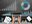# Is a fund's performance calculated after deducting all expenses?

Read to know how Value Research arrives at the performance of a particular fund on its websiteThe calculation of a fund's performance on our website Value Research Online is based on the growth in the fund's net asset value (NAV). NAV is calculated after taking out the expenses. So what is a NAV and how is it calculated? If we look at the formula for NAV, we'll understand it better.

So as depicted in the formula above, NAV is the total market value of all the shares held in a portfolio, including cash. Liabilities or expenses are deducted and it is then divided by the total number of units outstanding. When we are subtracting the liabilities from the assets, we take off all the expenses the fund incurs, including the management fees. So we are left with net assets. This exercise is done by the fund houses on a daily basis to derive the NAV of their respective funds. Whenever we are calculating returns generated by a mutual fund scheme, it is based on these daily NAVs published by the respective fund houses. For example, let's say the NAV of a fund is Rs 12 as of today and was Rs 10 exactly a year back. So the one-year return would be 20 per cent, as the NAV has appreciated by 20 per cent. Likewise, if the NAV would have depreciated from Rs 12 to Rs 10, the return generated by the fund over the last year would have been -20 per cent. When the returns for more than a year are calculated, compounded annualised growth rate (CAGR) is considered.

This is how the fund's performance is calculated. As the NAV itself is net of expenses, the returns too are net of the expenses.

What is NAV in mutual funds?

How to assess the performance of a mutual fund?

## Recommended Stories

Should you look at mutual fund size (AUM) before investing?

##### 3 min read •By Ravi Banagere

Should a retiree invest in a technology fund?

How to consolidate your mutual fund portfolio?

Knowledge vs guesswork

##### 5 min read •By Dhirendra Kumar

The three 'S' in mutual funds: SIP, STP, SWP

##### 4 min read •By Ravi Banagere

International mutual funds get some breather